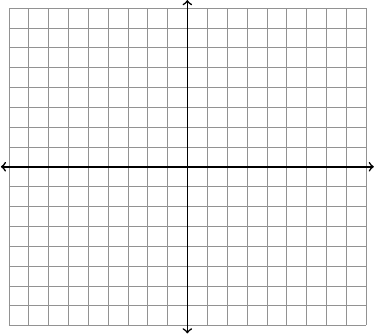Get 2 days of premium access
Class Notes (1,000,000)
US (430,000)
UC-Irvine (10,000)
MATH (1,000)
MATH 2D (200)
Lecture 10

# MATH 2D Lecture Notes - Lecture 10: Parametric Equation, Unit VectorExamPremium

Department
Mathematics
Course Code
MATH 2D
Professor
WILKINSON, J
Lecture
10

This preview shows pages 1-2. to view the full 8 pages of the document.Math 2D Practice Midterm 1
1. Consider the parametric curve x(t) = 1 + 2 sin(t) and y(t) = 3 4 cos(t)
(a) Find the rectangular form of this curve by eliminating the parameter.
(b) Sketch the graph of the curve on the axes below.
(c) Set up the integral representing the arc length of the curve for 0 t2π

Only pages 1-2 are available for preview. Some parts have been intentionally blurred.

Subscribers Only
2. For the vectors ~a = [3,2,4],~
b= [1,2,1],~c = [3,1,3] calculate:
~a + 2~
b4~c ~a ·~
b+~c~c ×~
k
Find a unit vector in the direction of ~a
What is the angle between ~
band ~c?
###### You're Reading a Preview

Unlock to view full version

Subscribers Only

Only pages 1-2 are available for preview. Some parts have been intentionally blurred.

Subscribers Only
3. (a) Find the equation plane containing the point (1,0,5) and the line L(t) = [1, t 1, t + 2]
(b) Find the distance between the planes 4xy+z= 2 and 4xy+z= 14
###### You're Reading a Preview

Unlock to view full version

Subscribers Only